Home > CAAC > Chapter 7 > Lesson 7.3.2 > Problem7-96

7-96.
1.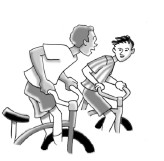Dean and Carlos decided to hold their own race. Dean estimates that he rides 3 meters every 4 seconds and wants a 5-meter head start. Carlos will ride 1 meter per second. Homework Help ✎

1. How many meters does Dean ride each second?

2. On one set of axes, graph and label lines to represent each rider's distance from the starting line. Find the equation for each rider.

3. Use the equations you wrote to determine when Carlos and Dean will be the same distance from the starting line.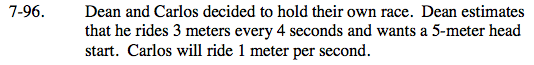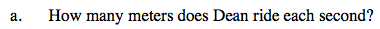Use a proportion to solve the problem.

$\frac{3}{4}=\frac{x}{1}$

x = 0.75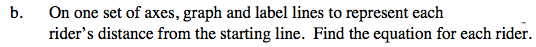Write the equations for each rider based on the given information.
Dean: y = 0.75x + 5
Carlos: write a similar equation.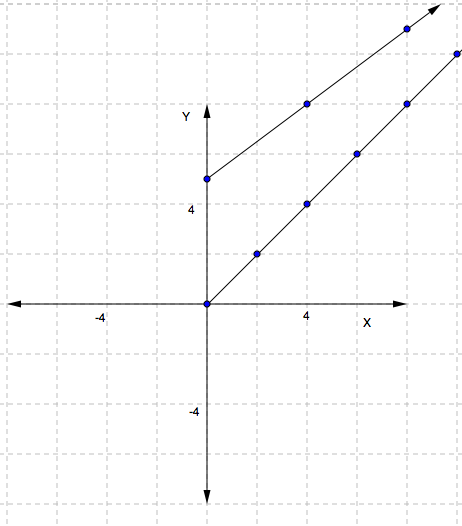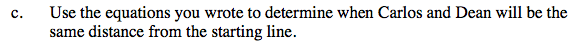Set the equations equal to each other and solve for x. Then substitute x back into one
of the original equations to solve for the distance from the starting line.